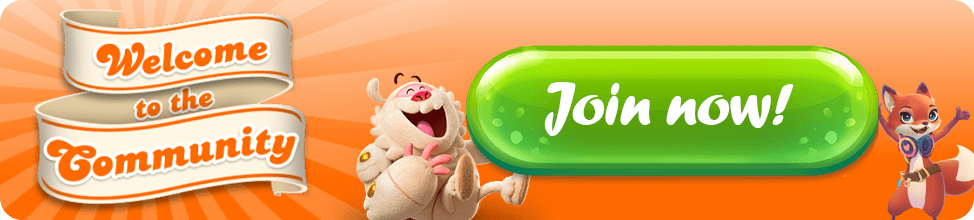Become Member of the Month and win 200 Gold bars!

(FINISHED) Are you the Community Mastermind and can crack the code?

• Greetings to all of the Players!

Finally, I got the code, which could be the right one, but I still have doubts regarding few quests.
11+17+22+19+28+18+25+28+30+20+22+21+30+25=316.

We all will find it out on July 14! Good luck 💰💰💰
• 316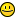• 315 is my answer
• 317 I think!
Fun games - thanks.
•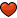My answer is 316.
• 317 and no more levels 😔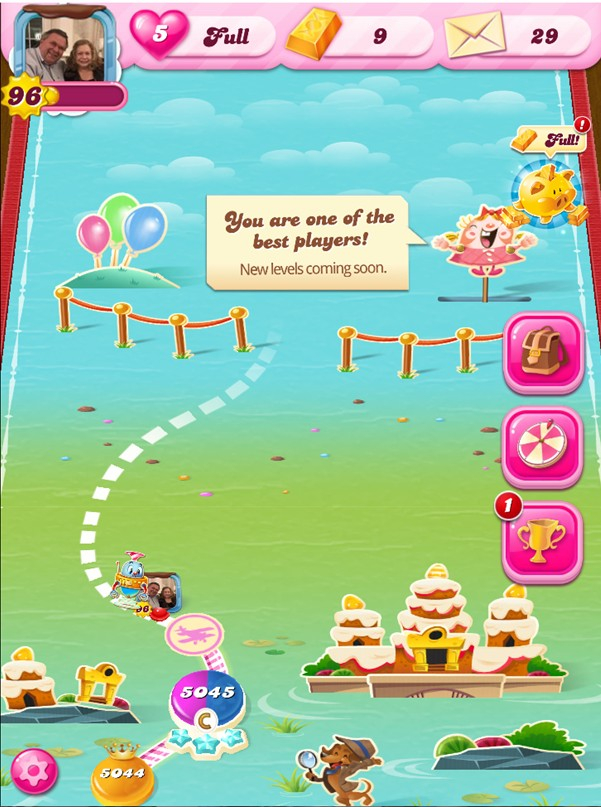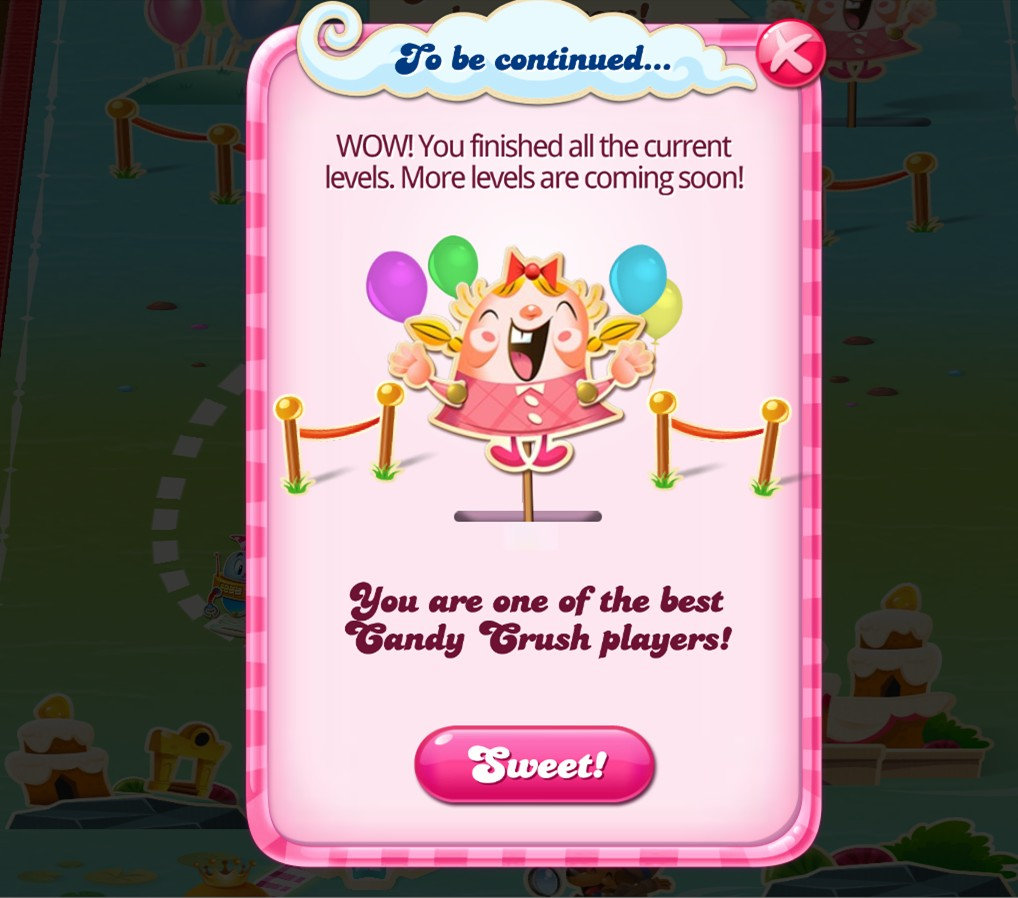•  My total is:     316

22+18+28+17+11+25+28+30
+19+20+22+25+21+30
=316

•316
• The final code to become Community MasterMind is *drumroll plz🥁🥁🥁🥁🥁🥁🥁🥁🥁🥁🥁🥁🥁🥁🥁🥁🥁🥁🥁
314

**Im probably wrong so I wouldn’t copy 🤣

GOOD LUCK EVERYONE 💞
• Hello @Crazy Cat Lad

1) Alpha Betty = 22
2) Blossom Blast = 18
3) Bubble Witch = 28
4) Candy Crush = 17
5) Candy Soda = 10
6) Candy Jelly = 26
7) Candy Friends = 28
8) Diamond Diaries = 30
9) Farm Heroes = 19
10) Papa Pear = 20
11) Pet Rescue = 22
12) Pet Puzzle = 25
13) Pyramid Solitaire = 21
14) Shuffle Cats = 30

---> So, my Total Tally is = 316 final answer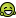This discussion has been closed.

Howdy, Stranger!

It looks like you're new here. If you want to get involved, click one of these buttons!